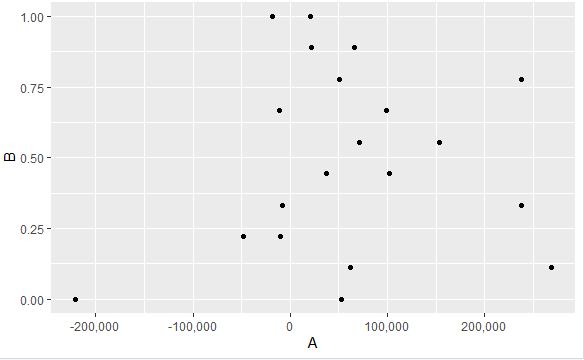2 views

I'm using R and ggplot to draw a scatterplot of some data, all is fine except that the numbers on the y-axis are coming out with computer-style exponent formatting, i.e. 4e+05, 5e+05, etc. This is obviously unacceptable, so I want to get it to display them as 500,000, 400,000, and so on. Getting a proper exponent notation would also be acceptable.

The code for the plot is as follows:

p <- ggplot(valids, aes(x=Test, y=Values)) +

geom_point(position="jitter") +

facet_grid(. ~ Facet) +

scale_y_continuous(name="Fluorescent intensity/arbitrary units") +

scale_x_discrete(name="Test repeat") +

stat_summary(fun.ymin=median, fun.ymax=median, fun.y=median, geom="crossbar")

Any help much appreciated.

by

You can set the labels argument to comma from the scales package to format the axis text labels.

library(scales)

scale_y_continuous(name="Fluorescent intensity/arbitrary units", labels = comma)

For example:

library(scales)

x <- rnorm(20) * 100000

y <- seq(0, 1, length = 10)

df <- data.frame(A = x,

B = y)

ggplot(df,aes(x=A, y=B)) +

geom_point() +

scale_x_continuous(labels = comma)

Output: